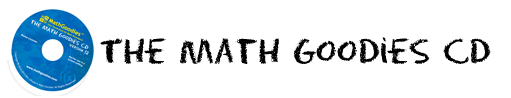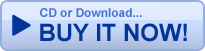# FREE Math Lessons & Math Worksheets from

## FREE Math Help With Interactive Math Lessons, Math Worksheets, Math Games and Fun Puzzles.

Topics such as: Circumference and Area of Circles; Median in Math; Symbolic Logic; Percentages and Probability; Geometry; Set Theory; and so much more!

Feature of the week
Lessons Integer Properties and Arithmetic
Worksheets Integer Worksheets
Math Puzzles Integer Vocabulary Puzzles
Math Videos Basic Properties of Integers
Game Integer Games (4 of them)
Math Award Certificate Build Your Own Template!Our CD has 168 in-depth math lessons organized into instructional units. For each unit, there is a corresponding set of worksheets and puzzles and learning games.